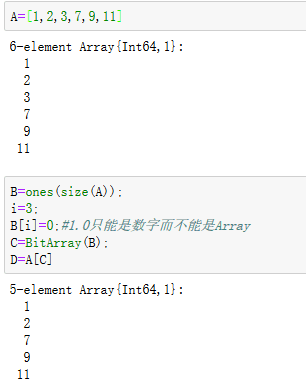# 怎么删除Array里面指定元素（或者Object）

``````mutable struct A
a

function A(a)
new(a)
end
end

a=Array{A,1}(undef,0)
A1=A(1)
A2=A(2)
A3=A(3)
A4=A(4)
A5=A(5)

push!(a, A1)
push!(a, A2)
push!(a, A3)
push!(a, A4)
push!(a, A5)

``````非常感谢！filter!(x->x!==A3, a)

`````` length(a) == length(filter!(x->x!==A3, a))
``````